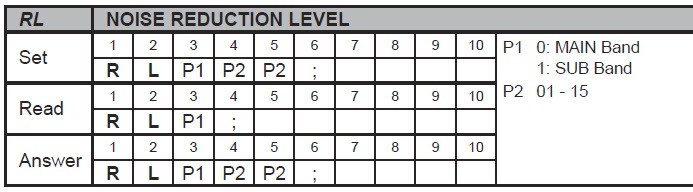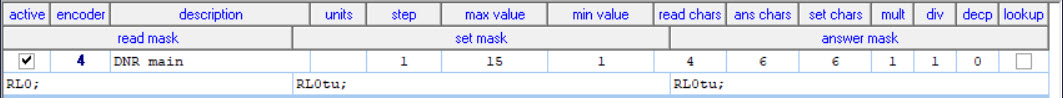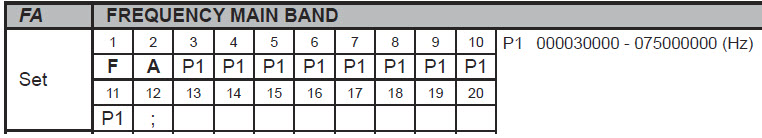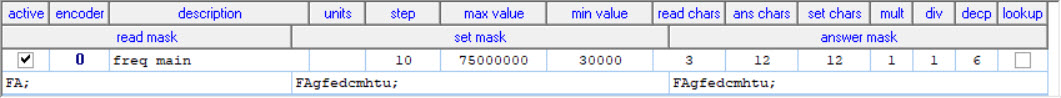EncoderCAT - Command masks examples   (Not used for Icom CI-V.)

The examples are from the Fdx101D. Other Yaesu radios have a similar CAT system.

A example from the FTdx101D CAT manual -  Noise Reduction Level.

The CAT manual entry is below. There are two identical receivers, MAIN and SUB.

(Note that the terminating semicolon is part of the command)For a change from value 6 to 7:

• MAIN  -  read = "RL0;"    answer  = "RL006;"   set = "RL007;"
• SUB   -  read = "RL1;"    answer  = "RL106;"   set = "RL107;"

In   MAIN.Answer  above:     "RL0" is the command and '"06' is the variable data.

xCAT configuration for main receiver noise reduction level  'RL0'• "RL0" are fixed as the first three characters.
• '"tu"  indicates that the fourth and fifth characters are variable tens and units.

Further example:   Main frequency

Yaesu CAT manual states:xCAT configuration for main frequency controlThe mask string is:  FAgfedcmhtu;  which represents numbers 0 - 999 999 999    ie: 0 - 999 Mhz

The FTdx101D range is 30khz to 75Mhz - so this determines the max and min settings, 75000000 and 30000.

I have chosen a step of 10Hz.

The decp (decimal places) setting of 6 provides an OLED display in Mhz   eg:   14.345700

I have provided this as an example of configuration and to prove that EncoderCAT can control main frequency.

I do not use EncoderCAT for rig tuning.

Main frequency control really needs a non-notched, high resolution encoder.

List of 'variable' characters

I have defined a small list of lower case characters that represent variable data.
All other characters outside this list are interpreted as fixed.

The characters are g, f, e, d, c, m, h, t, u   (u = units,   t= tens, h = hundreds  etc)

There is also s  = sign - representing  '-'  or  '+'

The codes are detailed below in an extract from EncoderCAT C source code:

EncoderCAT codes for character interpretation:

For decimal digit ascii characters, ie:  '0'  to  '9'

switch(mb)

{

case 'g': mult = 100000000; break;        // g = hundred millions

case 'f': mult = 10000000; break;        // f = ten millions

case 'e': mult = 1000000; break;        // e = millions

case 'd': mult = 100000; break;        // d = hundred thousands

case 'c': mult = 10000; break;                // c = ten thousands

case 'm': mult = 1000; break;                // m = thousands

case 'h': mult = 100; break;                // h = hundreds

case 't': mult = 10; break;                // t = tens

case 'u': mult = 1; break;                  // u = units

}

// Also    s represents sign '-' or '+'